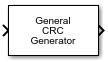# General CRC Generator

Generate CRC code bits according to generator polynomial and append to input data frames

• Library:
• Communications Toolbox / Error Detection and Correction / CRC

•## Description

The General CRC Generator block generates cyclic redundancy check (CRC) code bits for each input data frame and appends them to the frame. For more information, see CRC Generator Operation.

## Ports

### Input

expand all

Input signal, specified as a binary column vector. The length of the input frame must be a multiple of the value of the Checksums per frame parameter.

Data Types: `double` | `Boolean`

### Output

expand all

Output codeword frame, returned as a binary column vector that inherits the data type of the input signal. The output contains the input data frames with the CRC bit sequences appended to them.

The length of the output frame is m + k * r, where m is the size of the input frame, k is the number of checksums per frame, and r is the degree of the generator polynomial.

## Parameters

expand all

Generator polynomial for the CRC algorithm, specified as one of the following:

• A polynomial character vector such as ```'z^3 + z^2 + 1'```.

• A binary row vector that represents the coefficients of the generator polynomial in order of descending power. The length of this vector is (N+1), where N is the degree of the generator polynomial. For example, ```[1 1 0 1]``` represents the polynomial x3+ z2+ 1.

• An integer row vector containing the exponents of z for the nonzero terms in the polynomial in descending order. For example, `[3 2 0]` represents the polynomial z3 + z2 + 1.

Some commonly used generator polynomials include:

CRC methodGenerator polynomial
CRC-32`'z^32 + z^26 + z^23 + z^22 + z^16 + z^12 + z^11 + z^10 + z^8 + z^7 + z^5 + z^4 + z^2 + z + 1'`
CRC-24 `'z^24 + z^23 + z^14 + z^12 + z^8 + 1'`
CRC-16 `'z^16 + z^15 + z^2 + 1'`
Reversed CRC-16`'z^16 + z^14 + z + 1'`
CRC-8`'z^8 + z^7 + z^6 + z^4 + z^2 + 1'`
CRC-4 `'z^4 + z^3 + z^2 + z + 1'`

Example: `'z^7 + z^2 + 1'`, ```[1 0 0 0 0 1 0 1]```, and `[7 2 0]` represent the same polynomial, p(z) = z 7 + z 2 + 1.

Initial states of the internal shift register, specified as a binary scalar or a binary row vector with a length equal to the degree of the generator polynomial. A scalar value is expanded to a row vector of equal length to the degree of the generator polynomial.

Select to use the direct algorithm for CRC checksum calculations. When cleared, the block uses the non-direct algorithm for CRC checksum calculations.

For more information on direct and non-direct algorithms, see Error Detection and Correction.

Select to flip the input data on a bytewise basis before entering the data into the shift register. When Reflect input bytes is selected, the input frame length divided by the value of the Checksums per frame parameter must be an integer and a multiple of `8`. When Reflect input bytes is cleared, the block does not flip the input data.

Select to flip the CRC checksums around their centers after the input data are completely through the shift register. When Reflect checksums before final XOR is cleared, the block does not flip the CRC checksums.

Final XOR, specified as a binary scalar or a binary row vector with a length equal to the degree of the generator polynomial. The XOR operation runs using the value of the Final XOR parameter the CRC checksum before appending the CRC to the input data. A scalar value is expanded to a row vector of equal length to the degree of the generator polynomial. A setting of `0` is equivalent to no XOR operation.

Number of checksums calculated for each frame, specified as a positive integer.

## Block Characteristics

 Data Types `Boolean` | `double` Multidimensional Signals `no` Variable-Size Signals `yes`